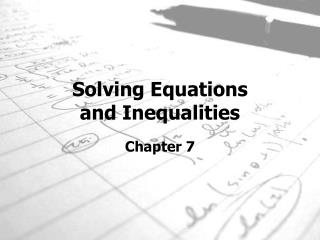DownloadDownload PresentationSolving Equations and Inequalities

# Solving Equations and Inequalities

Télécharger la présentation## Solving Equations and Inequalities

- - - - - - - - - - - - - - - - - - - - - - - - - - - E N D - - - - - - - - - - - - - - - - - - - - - - - - - - -
##### Presentation Transcript

1. Solving Equations and Inequalities Chapter 7

2. Solving Two-Step Equations Section 7-1

3. How to Solve a Two-Step Equation • One goal in solving an equation is to have only variables on one side of the equal sign and numbers on the other side of the equal sign. • The other goal is to have the number in front of the variable equal to one. • The variable does not always have to be x. These equations can make use of any letter as a variable.

4. How to Solve Two-Step Equations continued… The most important thing to remember in solving a linear equation is that whatever you do to one side of the equation, you MUST do to the other side. • So if you subtract a number from one side, you MUST subtract the same value from the other side. You will see how this works in the examples.

5. Solving Two-Step Equations continued… Solving a two-step equation requires the same procedure(s) as a one-step equation. However, the order in which the procedures are done makes a difference. Do the inverse operation for addition or subtraction first. Do the inverse operation of multiplication or division last. Inverse The operation that reverses the effect of another operation.

6. Solving Multi-Step Equations Section 7-2

7. Solving the Equation Step 1: You need to get the first term with the variable by itself. So you need to “UNDO” or get rid of -9. To do this, you do the OPPOSITE of what is being done. You are currently SUBTRACTING 9; the OPPOSITE is ADDITION. What you do to one side, you MUST do to the other. So you will ADD 9 to both sides. Step 2: The variable is still not by itself, so you need to do the OPPOSITE of what is being done. 3 is being multiplied to X and the OPPOSITE of multiply is DIVIDE. So you DIVIDE both sides by 3. The X is finally alone which means you have completed the equation. Solve 3x – 9 = 33

8. Finding Consecutive Integers • The definition of consecutive is “following one another in uninterrupted intervals.” • When you count by 1’s from any integer, you are counting consecutive integers. 1, 2, 3, 4 -5, -4, -3 The sum of three consecutive integers is96 Let n = the least integer. Then n + 1 = the second integer, and n + 2 = the third integer. n + n + 1 + n + 2 = 96 Four consecutive integers Three consecutive integers

9. Steps for Solving a Multi-Step Equation Step 1: Use the Distributive Property, if necessary. Step 2: Combine like terms. Step 3: Undo addition or subtraction. Step 4: Undo multiplication or division. Let’s Practice

10. To clear a fraction from an equation, you multiply both sides of the equation by the denominator. Examples 4x=12 2n-6=22 5 3 -7k+14=-21 1x+3=2 10 4 Solving Multi-Step Equations with Fractions

11. How to Clear Equations of Fractions TO SOLVE AN EQUATION WITH fractions, we transform we transform it into an equation without fractions – which we know how to solve. The technique is called clearing of fractions. Example 1 Solve for x: x + x − 2 = 6 3       5  Multiply both sides of the equation--every term--by the LCM of denominators.  Every denominator will then cancel.  We will then have an equation without fractions. The LCM of 3 and 5 is 15.  Therefore, multiply every term on both sides of the equal sign by 15. Each denominator will now cancel into 15--that is the point--and we have the following simple equation that has been "cleared" of fractions. Caution! Be sure the distributive law is used to multiply all of the terms by 15.

12. How to Clear Equations of Decimals To clear an equation of decimals, we count the greatest number of decimal places in any one number. If the greatest number of decimal places is 1, we multiply both sides by 10; if it is 2, we multiply by 100; and so on. Example 2 Solve:16.3 - 7.2y = -8.18 Solution The greatest number of decimal places in any one number is two. Multiplying by 100 will clear all decimals.

13. Quick Check Solve each equation: • -7 + y = 1 b. 1b – 1 = 5 12 6 3 6 c. 1.5x – 3.6 = 2.4 d. 1.06p – 3 = 0.71

14. Write an Equation Section 7-4